# Power Of Matrices

The matrices can be multiplied to get product matrix and also they demonstrate all other mathematical properties. The power of matrices is another mathematical property of matrix where matrix is raised to a power using an exponent. This brings another question, does the exponent laws applies to matrices or not ? what type of matrices qualifies to be raised to some power ? What about common mathematical identities that involve matrices and power of matrices.

### Exponents or Power of a Number

Exponent or power is a number which tell us how many times a number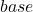should multiplied by itself. If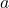represents a base and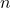is its power, then its written as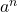which meansFigure 1 – a is raised to power n which means ‘a’ multiplied n times to get a final product

Similarly, a square matrix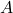and an integeris given, then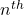power ofis defined as product matrix obtained by multiplyingby itselftimes.

A^n = A \times A \times A \times ... \times A \hspace{5px}(n \hspace{5px}times)

Note that the matrixis

• a square matrix
• and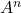is a product matrix of same order.

The exponents have their own algebra which is given as follows.

Basic Laws of Exponents

The basic laws of exponents applied to any real number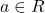and these are

•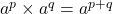•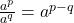•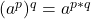•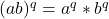We need to find out whether these laws applies to square matrices or not. Let us verify this claim with examples.

Example Proof #1

Supposeis a square matrix of order 2 x 2.

\begin{aligned}
&A = \begin{bmatrix}1 & 2\\2 & 3\end{bmatrix}\\\\
&A^2 = \begin{bmatrix}1 & 2\\2 & 3\end{bmatrix} \times \begin{bmatrix}1 & 2\\2 & 3\end{bmatrix} = \begin{bmatrix}1+4 & 2+6\\2+6 & 4+9\end{bmatrix} = \begin{bmatrix}5 & 8\\8 & 13\end{bmatrix}\\\\
&A^3 = \begin{bmatrix}5 & 8\\8 & 13\end{bmatrix} \times \begin{bmatrix}1 & 2\\2 & 3\end{bmatrix} = \begin{bmatrix}5+16 & 10+24\\8+26 & 16+39\end{bmatrix} = \begin{bmatrix}21 & 34\\34 & 55\end{bmatrix}\\\\
&A^2 \times A^3 = A^{2 + 3} = A^{5}\\\\
&A^2 \times A^3 = \begin{bmatrix}5 & 8\\8 & 13\end{bmatrix} \times \begin{bmatrix}21 & 34\\34 & 55\end{bmatrix} = \begin{bmatrix}105+272 & 170+440\\168+442 & 272+715\end{bmatrix} = \begin{bmatrix}377 & 610\\610 & 987\end{bmatrix}\\\\
&
\end{aligned}

Also,

\begin{aligned}
&A^5 = \begin{bmatrix}377 & 610\\610 & 987\end{bmatrix}
\end{aligned}

Therefore, both side of the equation is equal.

Example Proof #2

There is not concept of division in matrix, however, you can divide element of matrix by multiplying it with an inverse value which is same as dividing the element. Inverse of a matrix is covered in the next lesson.

Ifis a square matrix of order 2 x 2. Then,

\begin{aligned}
A = \begin{bmatrix}1 & 2\\2 & 3\end{bmatrix}
\end{aligned}

Therefore,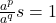is not possible, but ifis an invertible matrix then,

\begin{aligned}
A.A^{-1} = I
\end{aligned}

Where,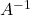is inverse of the matrixof same order and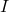is called the identity matrix of same order.

Example Proof #3

The power of power of a matrix is a product matrix with exponents multiplied. Ifis a square matrix raised to power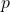and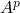is also raised to the power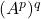, then the resultant product matrix is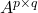of same order.

Letbe a square matrix with order 2 x 2.

\begin{aligned}
A = \begin{bmatrix}1 & 2\\2 & 3\end{bmatrix}
\end{aligned}

Then,

\begin{aligned}
&(A^2)^3 = A^{2 \times 3} = A^6\\\\
&(A^2)^3 = \begin{bmatrix}5 & 8\\8 & 13\end{bmatrix}^3\\\\
&A^{2 \times 3} = \begin{bmatrix}5 & 8\\8 & 13\end{bmatrix} \times \begin{bmatrix}5 & 8\\8 & 13\end{bmatrix} \times \begin{bmatrix}5 & 8\\8 & 13\end{bmatrix}\\\\
&A^{2 \times 3} = \begin{bmatrix}89 & 144\\144 & 233\end{bmatrix} \times \begin{bmatrix}5 & 8\\8 & 13\end{bmatrix}\\\\
&A^{2 \times 3} = \begin{bmatrix}445 + 1152 & 712 + 1872\\720 + 1864 & 1152+3029\end{bmatrix}\\\\
&A^{2 \times 3} = \begin{bmatrix}1597 & 2584\\2584 & 4184\end{bmatrix}\\\\
&Also,\\\\
&A^6 = \begin{bmatrix}1597 & 2584\\2584 & 4184\end{bmatrix}
\end{aligned}

Example Proof #4

The product of defined matrices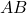raised to poweris equal to product of powers of individual matricesandraised to the power.

\begin{aligned}
&(AB)^p = A^p \times B^p\\\\
\end{aligned}

We can rewrite the equation as

\begin{aligned}
&(AB)^p = (AB)(AB)(AB)... p-times\\\\
&(AB)^p = A(BA)(BA)B ... p-times
\end{aligned}

But we know thatis not commutative.

\begin{aligned}
AB \ne BA
\end{aligned}

Therefore,

\begin{aligned}
(AB)^p \ne A^p \times B^p
\end{aligned}

### Why Only Square Matrix ?

Only square matrix is suitable for exponents or to be raised to some powers because of two reasons.

• Non-Square or Singular matrices are not defined. Ifis a non-square matrix of order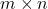thenis not possible because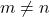where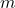is row andis column of matrix.
• When we need to take inverse which is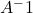, the matrix must be a square. Singular matrices are not invertible.

Example Proof #5

Letbe a non-square matrix with order. Letbe an non-negative integer whose value is 2.

\begin{aligned}
&A = \begin{bmatrix}1 & 4 & 2\\2 & 0 & 1\end{bmatrix}\\\\
\end{aligned}

Let us try to obtain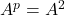, then we observe that it is not possible.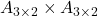is undefined where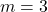and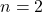. This is becauserequired for multiplication of matrices.

Therefore, a matrix cannot be raised to power unless it is a square matrix.

Example Proof #6

Another reason to use square matrix with power is to find inverse matrix. Ifis a matrix that is invertible and we wish to find the inverse matrixsuch that

\begin{aligned}
A.A^{-1} = A^{-1}.A = I_{n \times n}
\end{aligned}

The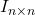represents an identity matrix whose main diagonals are 1 and rest of the entries are 0. It is a square matrix. Therefore,andmust be square matrices.

In the next section, we will explore whether matrices complies with common algebraic identities or not.

### Common Algebraic Identities And Square Matrices

The standard algebraic identities are true for any value of variables. Instead of numbers, we will use square matrix to prove these identities holds for matrices too.

•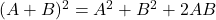•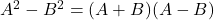•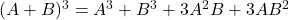•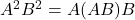Order Of Multiplication

In matrix multiplication, the order of multiplication is very important because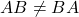which is even true for square matrices.

If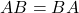, then any one of the following is true.

• The matrix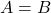.
• Eitheroris identity matrix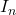.
• Eitheroris zero or null matrix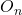.
• Theis inverse ofor the matrixis inverse of.

Let us now verify the common algebraic identities with matrices as variables.

Example Proof #7

We check the following identity :.

Letandbe square matrices of order 2 x 2.

\begin{aligned}
&(A + B)^2 = (A + B)(A + B)\\\\
&= A^2 + AB + BA + B^2
\end{aligned}

But, therefore,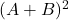not possible.

Example Proof #8

We will verify the claim: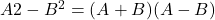Letandbe square matrix of order 2 x 2.

\begin{aligned}
&A^2 - B^2 = (A + B)(A - B)\\\\
&
\end{aligned}

We can write the right-hand side as,

\begin{aligned}
&= A^2 - AB + BA - B^2
\end{aligned}

But we know that, therefore, above identity is false.

Example Proof #9

We check the identity:.

Letandbe square matrices of order 2 x 2. We multiply (A + B)^3, we get following results.

\begin{aligned}
&(A + B)(A + B)(A + B)
\end{aligned}

Since, we know thatis not possible. Therefore,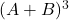is also false.

Example Proof #10

We now verify the identity :Letandbe square matrices and single both are defined. We get following equation from \$A^2B^2.

\begin{aligned}
&A^2B^2 = A.A.B.B
\end{aligned}

We get,

\begin{aligned}
A^2B^2 = A(A.B)B
\end{aligned}

Therefore, the identity is true because the order of multiplication is same.